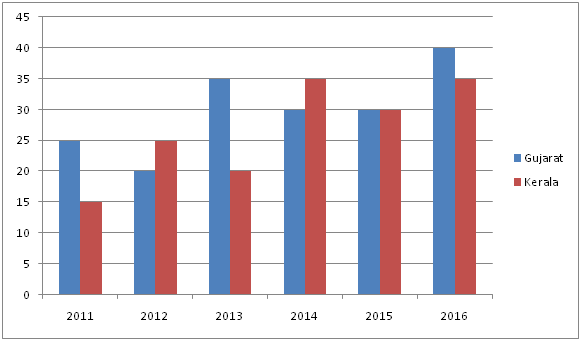# LIC AAO/SBI PO Prelims Quantitative Aptitude Questions 2019 (Day-08)

Dear Aspirants, Our IBPS Guide team is providing new series of Quantitative Aptitude Questions for LIC AAO/SBI PO 2019 so the aspirants can practice it on a daily basis. These questions are framed by our skilled experts after understanding your needs thoroughly. Aspirants can practice these new series questions daily to familiarize with the exact exam pattern and make your preparation effective.

[WpProQuiz 5590]

### Click Here for SBI PO Pre 2019 High-Quality Mocks Exactly on SBI Standard

Directions (Q. 1 – 5): What value should come in place of question mark (?) in the following questions?

1) 2401 – (42875)% of 420 + √(1296)% of  600 = ?

a) 62

b) 64

c) 78

d) 76

e) None of these

2) √1024 ÷ 16 × 82 + 254 =? – 5016 ÷ 11

a) 756

b) 838

c) 912

d) 964

e) None of these

3) (32)2 × (64)1/3 × (48)2 ÷ (27 × (12)2) = 2?

a) 9

b) 7

c) 8

d) 11

e) None of these

4) 4 (7/9) of (9873 – 3195) + 368 =? + 2800 – 34 % of 800

a) 31258

b) 33524

c) 29746

d) 27352

e) None of these

5) 3 (½) + 5 (7/8) – 6 (¼) =? – 4 (¾) + 2 (1/8)

a) 6 (5/6)

b) 4 (¼)

c) 7 (½)

d) 5 (¾)

e) None of these

Directions (Q. 6 – 10): Study the following information carefully and answer the given questions.

The following bar graph shows the percentage of growth in the population of two states.6) Find the average percentage growth of population of Gujarat in all the given years together?

a) 28

b) 26

c) 30

d) 24

e) None of these

7) If the population of Kerala in the year 2013 was 2.3 lakhs, then find the population of Kerala in the year 2011?

a) 1.6 lakhs

b) 1.4 lakhs

c) 1.8 lakhs

d) 1.5 lakhs

e) None of these

8) If the population of Gujarat in the year 2014 was 5.6 lakhs and the population of Kerala in the year 2014 was 4 lakhs, then find the ratio of population of Gujarat to that of Kerala in the year 2016?

a) 135: 189

b) 567: 352

c) 15: 8

d) 182: 135

e) None of these

9) The percentage growth of population of Kerala in the year 2011 and 2012 together is approximately what percentage more than the percentage growth of population of Gujarat in the year 2013?

a) 20 %

b) 15 %

c) 10 %

d) 25 %

e) 30 %

10) Find the difference between the total population of Gujarat in 2014 to that of total population of Kerala in 2015, if the total population of Gujarat in 2015 is 5.2 lakhs and the population of Kerala in 2016 is 6.5 lakhs?

a) 1 lakh

b) 1.5 lakhs

c) 2 lakhs

d) 2.25 lakhs

e) None of these

Direction (1-5) :

∜2401 – ∛(42875)% of 420 + √(1296)% of  600 = x

7 – (35/100)*420 + (36/100)*600 = x

X = 7 – 147 + 216 = 76

√1024 ÷ 16 × 82 + 254 = x – 5016 ÷ 11

(32/16)*8*8 + 254 = x – (5016/11)

128 + 254 = x – 456

X = 128 + 254 + 456 = 838

(32)2 × (64)1/3 × (48)2 ÷ (27 × (12)2) = 2x

(32*32*4*48*48)/ (27 * 12 * 12) = 2x

(25 * 25 * 26)/ 27 = 2x

216 – 7 = 2x

29 = 2x

X = 9

(43/9)*6678 + 368 = x + 2800 – (34/100)*800

31906 + 368 – 2800 + 272 = x

X = 29746

3 (½) + 5 (7/8) – 6 (¼) + 4 (¾) – 2 (1/8) = x

X = (3 + 5 – 6 + 4 – 2) (1/2 + 7/8 – ¼ + ¾ – 1/8)

X = 4 [(4 + 7 – 2 + 6 – 1)/8]

X = 4 (14/8) = 4 (7/4) = 5 (¾)

Direction (6-10) :

The average percentage growth in population of Gujarat all the given years together

= > (25 + 20 + 35 + 30 + 30 + 40)/6

= > 180/6 = 30

Let the population of Kerala in the year 2011 be x,

According to the question,

= > x*(115/100)*(125/100) = 2.3

= > x = (2.3*100*100)/(125*115)

= > x = 1.6 lakhs

The population of Gujarat in the year 2016

= > 5.6*(130/100)*(130/100)

The population of Kerala in the year 2016

= > 4*(135/100)*(130/100)

Required ratio = [5.6*(130/100)*(130/100)]: [4*(135/100)*(130/100)]

= > 36.4: 27

= > 364: 270

= > 182: 135

The percentage growth of population of Kerala in the year 2011 and 2012 together

= > 15 + 25 = 40 %

The percentage growth of population of Gujarat in the year 2013 = 35 %

Required % = [(40 – 35)/35]*100 = 14.28 % = 15 %

Let the population of Gujarat in 2014 and that of Kerala in 2015 be x and y respectively,

The total population of Gujarat in 2015 = 5.2 lakhs

X*(130/100) = 5.2

X = 5.2*(100/130) = 4 lakhs

The population of Kerala in 2016 = 6.5 lakhs

Y*(130/100) = 6.5

Y = 6.5*(100/130) = 5 lakhs

Required difference = 5 – 4 = 1 lakh